# CPP入门

### 输入&输出

C和CPP本身都没有提供输出和输入的专门语句,cin和cout是CPP中的标准输入输出流。类似C中使用printf和scanf需要包含<stdio.h>头文件，CPP中使用cout和cin需要包含头文件，并且使用命名空间 std。

``````#include <iostream>
using namespace std;
int main()
{
int num = 0;
//从键盘输入10
cin >> num;//将10读入num
cout << num << endl;//打印num
std::cout << num << std::endl;//如未使用命名空间std，则需要指定
return 0;
}``````

### 作用域运算符

``````#include <iostream>
using namespace std;
int a = 10;
void test()
{
int a = 20;//全局变量和局部变量同名，则局部优先级高于全局
cout << a << endl;//打印局部变量
cout << ::a << endl;//使用作用域运算符::打印全局变量
}``````

### 命名空间

CPP中引入了namespace的概念，大型工程中通常由不同的人进行代码的编写，因此为了避免标识符(变量名、函数名、类名等)相同而造成冲突引入此概念

``````#include <stdio.h>
using namespace std;
namespace A
{
int num = 10;
}
namespace B
{
int num = 20;
}
int main()
{
cout << A::num << endl;//10
cout << B::num << endl;//20
return 0;
}``````

1.namespace只能在全局范围内定义，即不能在函数内部定义

2.namespace可以嵌套定义

``````namespace A
{
int num = 10;
namespace B
{
int num = 20;
}
}
cout << A::num << endl; //10
cout << A::B::num << endl; //20``````

3.namespace是开放的，可以随时添加新成员

``````namespace A
{
int x = 10;
}
namespace A
{
int y = 20;
}``````

4.namespace可以存放函数，且函数可以在外部定义

``````namespace A
{
int num = 10;
void fun();
}
void A::fun()//外部定义需要加上作用域，否则为普通函数
{
cout << num << endl;
}``````

5.namespace可以无名，实际无用处

6.namespace可以取别名

``````namespace verylongspacename
{
int num = 100;
void fun()
{
cout << "verylongspacename" << endl;
}
}
int main()
{
namespace shortname = verylongspacename;
//可以通过两种形式使用
verylongspacename::fun();
shortname::fun();
return 0;
}``````

7.通过using使用namespace，并且可以指定namespace中的具体成员，如果出现冲突，采取就近原则

``````namespace A
{
int num = 10;
}
int main()
{
int num = 1000;
using namesapce A;
cout << num << endl;//出现冲突，采取就近原则，输出为1000
return 0;
}``````
``````namespace A
{
int X = 10;
int Y = 20;
}
int main()
{
using A::X;//使用namespace中的具体成员
cout << X << endl;//10
cout << Y	<< endl;//此句语法错误 正确写法为：cout << A::Y << endl;
int X = 20;
using A::X;
//如果使用namespace中的具体成员与局部变量冲突会造成编译错误 11和12行
return 0;
}``````

### 函数重载

``````#include <iostream>
using namespace std;
void fun(int x)
{
cout << "int" << endl;
}
void fun(int x,int y)
{
cout << "int int" << endl;
}
void fun(int x,double y)
{
cout << "int double" << endl;
}
/*

void fun(int x) ----> void fun_int(int x)
void fun(int x,int y) ----> void fun_int_int(int x)
void fun(int x,double y) ----> void fun_int_double(int x,double y)
*/
int main()
{
fun(10);//输出为:	int
fun(10,20);//输出为: int int
fun(10,9.99);//输出为: int double
return 0;
}``````

### CPP对比C的增强

1.全局变量检测增强

``````int a = 10;
int a;``````

2.对类型检测的增强

``````void fun(x)//C语言中缺省形参类型则 x 为 int 型
{

}
void fun(x)//C++中语法错误
{

}``````

3.类型转换的增强

``````typedef enum COLOR
{
RED,
BULE,
YELLOW
}COLOR;
COLOR mycolor = RED;
COLOR mycolor = 10;``````

4.结构体的增强

``````#include <iostream>
using namespace std;
struct DATA
{
int age;
char sex;
void fun(int age)//C中不能定义函数，CPP则可以,16行为调用方法
{
cout << age << endl;
}
};
int main()
{
struct DATA A;//C中不能缺省struct关键字
DATA B;//C++可以缺省struct关键字
A.fun(50);
return 0;
}``````

5.增加bool类型
bool类型大小为1字节，只有两个值，true和false
C中C99标准才有bool类型，在头文件stdbool.h中

6.三目运算符的增强
C中三目运算符的返回值的是数值，为右值，不能对其赋值
C++中三目运算符的返回值为变量本身，为左值，可以对其赋值

``````int x = 10,y = 20;
x > y ? x : y = 100;//在C中此行语法错误因为返回的是20，CPP中返回的是y的引用
cout << y << endl;//在CPP中输出为100``````

### const

const在C和CPP中的差异

``const int a = 10;``

(只有在const修饰局部变量的时候才能通过地址修改，局部变量内存空间在栈区，可读可写。而如果const修饰的是全局变量则无法修改，全局变量的内存空间在文字常量区，只可读)，修改后无论是通过变量名或者是地址访问都是修改后的值

``````#include <stdio.h>
int main()
{
const int a = 10;
a = 100;//错误
int *p = (int*)&a;
*p = 100;
printf("%d",a);//通过变量名访问为修改后的值100
printf("%d",*p);//通过地址访问为修改后的值100
return 0;
}``````

​ 在CPP中，const修饰全局变量，默认为内部链接，只能作用于当前文件，定义处加上extern可转换外部链接。系统不会为其开辟空间，而是放到符号表当中，

NAME VALUE
a 10

``````#include <iostream>
using namespace std;
int main()
{
const int a = 10;
int *p = (int*)&a;
*p = 100;
cout << a << endl;//通过变量名访问的是符号表的值，符号表的值不会修改，为10
cout << *p << endl;//通过地址访问的实际内存空间的值，为100
//
int c = 10;
const int b = c;//通过变量进行赋值，直接开辟内存空间
int *p = (int*)&b;
*p = 100;
cout << a << endl;//为100
cout << *p << endl;//为100
return 0;
}``````

​ 1.类型。const具有类型，编译器会对其进行类型安全检查，而define无类型，在预处理阶段就完成替换，不会进行类型检查
​ 2.作用域。const的作用域为定义其函数和复合语句内部；define的作用域为定义处到文件结束
​ 3.命名空间。const可以作为命名空间成员，而define不可以

### 引用

``````#include <iostream>
using namespace std;
int main()
{
int a = 10;
int& b = a;//b等价于a
b = 20;
cout << a << endl;//输出为20
return 0;
}``````

``````int num = 10;
int &ref = num;// 等价于 int* const ref = &num;``````

``````#include <iostream>
using namespace std;
int main()
{
//第一种方式
int arr = {1,2,3,4,5};
int (&my_arr) = arr;//[]优先级高于&，所以需要括号
//第二种方式
int arr = {1,2,3,4,5};
typedef int arr_five;//arr_five等价于int 
arr_five &my_arr = arr;
}``````

``````#include <iostream>
using namespace std;
void swap_by_point(int *x,int *y)//通过指针交换两个数
{
int tmp = *x;
*x = *y;
*y = tmp;
}
void swap_by_ref(int &a,int &b)//通过引用交换两个数
{
int tmp = a;
a = b;
b =	tmp;
}
int main()
{
int x = 10,y = 20;
swap_by_point(&x,&y);
swap_by_ref(x,y);
/*
为什么通过引用的方式传的是int类型，而函数形参是int&？
这是因为当函数形参为引用时，实参不是简单的传参，而是将实参绑定到形参上，也就是为引用进行初始化
*/
return 0;
}``````

``````#include <iostream>
using namespace std;
int& fun()
{
static int num = 10;
return num;
}
int main()
{
int &ret = fun();
cout << ret << endl;//10
return 0;
}``````

``````#include <iostream>
using namespace std;
int& fun()
{
static int num = 10;
return num;
}
int main()
{
fun() = 1000;
int &ret = fun();
cout << ret << endl;//1000
return 0;
}``````

(封装一个函数，从堆区开辟空间，赋值为”hello world”)

``````#include <iostream>
#include <stdlib.h>
#include <string.h>
using namespace std;
void by_point(char **str)//通过指针实现
{
*str = (char*)malloc(sizeof(char) * 10);
strcpy(*str,"hello world");
return;
}
void by_ref(char* &str)//通过引用实现
{
str = (char*)malloc(sizeof(char) * 10);
strcpy(str,"hello wrold");
return;
}
int main()
{
char *str = NULL;
by_point(&str);
by_ref(str);
return 0;
}``````

``void fun(const int &ref)``

``const int &ten = 10;``

### 缺省函数参数

CPP在声明函数原型时可以为一个或多个参数指定默认的参数值 ，在函数实参缺省的情况下则会 自动使用默认参数值

``````#include <iostream>
int fun(int x = 10,int y = 20)
{
return x + y;
}
int main()
{
cout << fun(100,200) << endl;//使用实参值，输出300
cout << fun() << endl;//实参缺省，使用形参默认值，输出30
cout << fun(20) << endl;//从左至右，缺省一个实参值，输出40
return 0;
}``````

``````void fun(int x,int y = 10)//合法，至少传一个实参值
void fun(int x,int y,int z = 20)//合法，至少传两个实参值
void fun(int x,int y = 10,int z)//非法，y设置了默认参数那么y后面的参数都必须有默认参数``````

``````int fun(int x = 10,int y = 20);
int fun(int x,int y)
{
return x + y;
}``````

``````void funA(int a);
void funA(int a,int b = 10);
funA(10);//此时无法确定调用哪个函数``````

### 占位参数

``````int fun(int x,int y,int)
{
return x + y;
}
fun(10,20,30);//必须为占位参数传值``````

### C和CPP混合编程

main.cpp

``````#include <iostream>
using namespace std;
int main()
{
return 0;
}``````

``````#ifndef __ADD_H

#if __cplusplus//如果是cpp文件
extern "C"
{
#endif

#if __cplusplus
}
#endif

``````int add(int x,int y)
{
return x + y;
}``````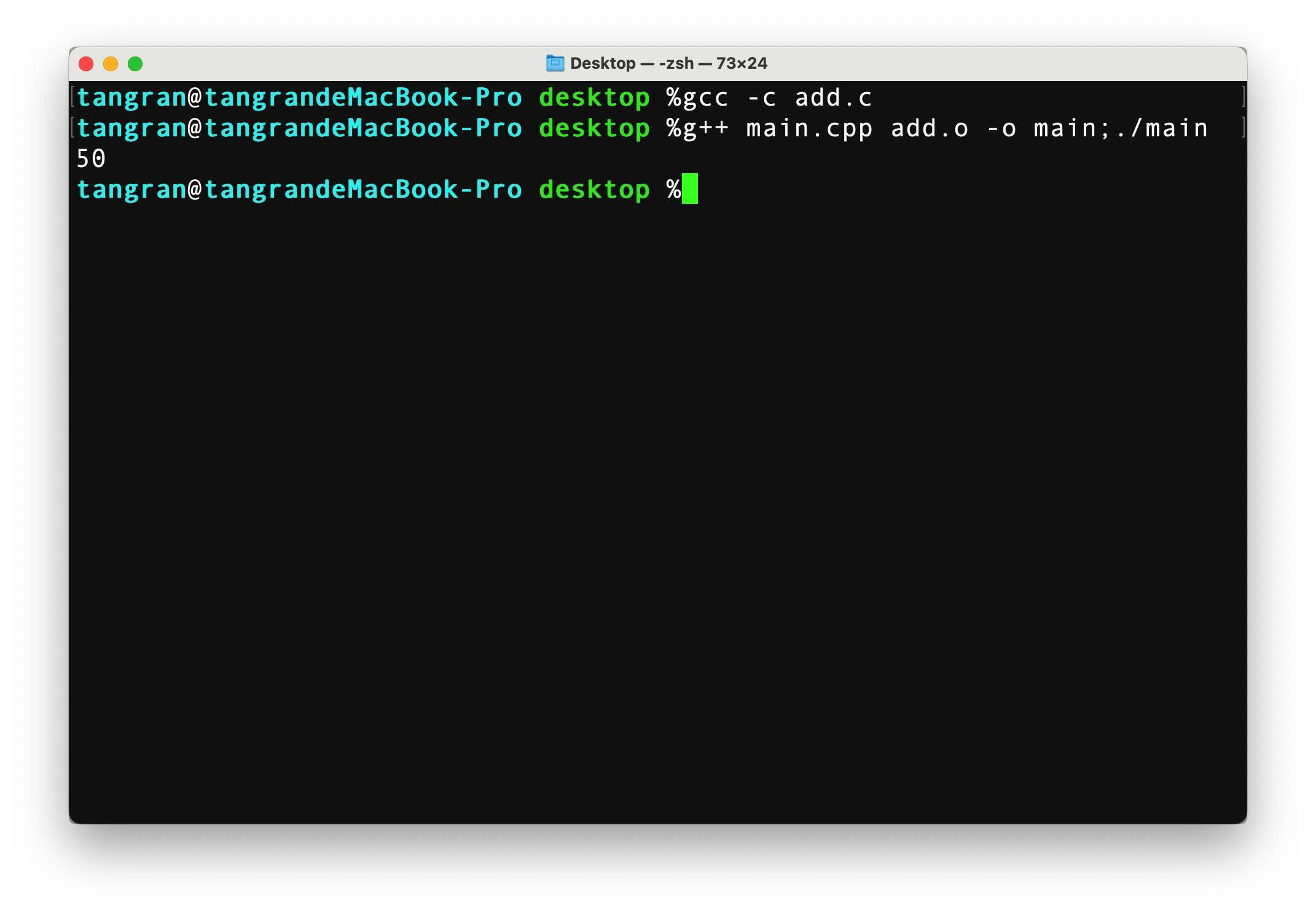### 类

``````class Person//类名
{
private://权限
string name;//属性
public://权限
void show()//方法
{
cout << "my name is " << name << "."<< end;
}
};``````

1. 访问数据的一致性。使得所有public接口均为函数，当操作类的时候，不必花时间去判断是函数还是变量。
2. 可执行权限的划分。如果将变量声明为public，可执行权限则为可读可写，但如果通过函数来间接操作，就能实现多种执行权限，如：只可读、只可写等。
3. 变量的合法性。一些变量具有限制，如年龄不可以是负数，因此可以通过在函数中实现安全判断，保证变量的合法性。

``````class Base;//前向声明，不完全类型
Base func(void);//不完全类型做返回值
void funb(Base);//不完全类型做参数
Base *p = NULL;//指向不完全类型的指针``````

Main.cpp

``````#include <iostream>
#include "person.h"
using namespace std;
int main()
{
Person a;
a.setAge(10);
cout << a.getAge() << endl;
return 0;
}``````

person.h

``````#ifndef __PERSON_H
#define __PERSON_H

class Person
{
private:
int age;
public:
void setAge(int n);
int getAge(void);
};

#endif//__PERSON_H``````

person.cpp

``````#include "data.h"
void Person::setNum(int n)//需要加上作用域，表明此函数为该类的成员函数，否则为普通函数
{
num = n;
}
int Person::getNum(void)
{
return num;
}``````

### 构造函数&析构函数

``````#include <iostream>
using namespace std;
class Test
{
private:
int num;
public:
Test()//无参构造函数
{
num = 0;
cout << "我是无参构造" << endl;
}
Test(int n)//有参构造函数
{
num = n;
cout << "我是有参构造" << endl;
}
~Test()//析构函数
{
cout << "我是析构函数" << endl;
}
Test(const Test &ob)//拷贝构造函数
{
cout << "我是拷贝构造" << endl;
}
};
void fun()
{
Test a(10);
}
int main()
{
cout << "start" << endl;
fun();
cout << "end" << endl;
return 0;
}``````

``````start

end``````

``````//无参构造(隐式)
Test a;
//无参构造(显式)
Test a = Test();
//有参构造(隐式)
Test a(10);
//有参构造(显式)
Test a = Test(10);
//隐式转换的方式(只能用于只有一个成员变量，不建议使用)
Test a = 10;
//匿名对象(生命周期为当前语句)
Test(10);``````

explicit关键字：

explicit关键字作用于只有一个成员变量的类构造函数，它表明该构造函数是显式而并非隐式的，防止编译器进行隐式转换。

``````class A
{
private:
int m_num;
public:
explicit A(int x):m_num(x) {}
};
A a(5);//✅
A a = 5;//❌``````

``````Test A(10);
//隐式
Test B(A);
//显式
Test B = Test(A);
//隐式转换
Test B = A;``````

``````#include <iostream>
#include <string.h>
#include <stdlib.h>
using namespace std;
class Person
{
private:
char *m_name;
public:
Person()
{
m_name = NULL;
}
Person(const char *name)
{
cout << "有参构造" << endl;
m_name = (char*)malloc(strlen(name) + 1);
if(m_name)
{
cout << "申请空间成功" << endl;
strcpy(m_name,name);
}
}
~Person()
{
cout << "析构函数" << endl;
if(m_name)
{
free(m_name);
m_name = NULL;
}
}
};
int main()
{
Person A("Lihua");
Person B(A);
return 0;
}``````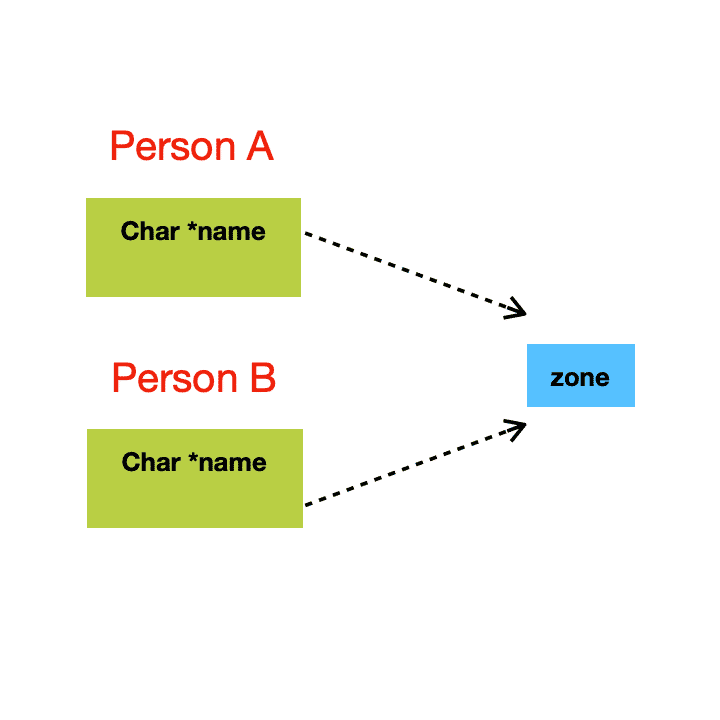``````Person(const Person &ob)//深拷贝
{
m_name = (char*)malloc(strlen(ob.m_name) + 1);
if(m_name)
{
strcpy(m_name,ob.m_name);
}
}``````

### 初始化列表

``````class Test
{
private:
int m_x,m_y,m_z;
public:
Test(int x,int y,int z):m_x(x),m_y(y),m_z(z)//初始化列表
{

}
//or
Test(int x,int y,int z)
:m_x(x),m_y(y),m_z(z)
{

}
}``````

``````class Test
{
int x,y;
public:
Test(int val):y(val),x(y){}
}``````

1. 使用初始化列表底层效率比函数体赋值更高。
``````#include <iostream>
using namespace std;
class Base
{
public:
Base()
{
cout << "无参构造" << endl;
}
Base(int x)
{
cout << "有参构造" << endl;
}
};
class Sub
{
private:
Base m_b;
public:
Sub()
{
m_b = Base(5);
}
};
int main()
{
Sub a;
return 0;
}``````

2.类中带有const成员变量、引用成员变量、自定义类型成员变量(类无默认构造函数)时必须使用初始化列表。初始化const和引用变量的唯一机会就是通过初始化列表。

### new&delete

new&delte和malloc&free的区别有以下几点：

1. malloc仅分配内存不会调用构造函数，free仅回收内存不会调用析构函数，而new&delete会。
2. new返回的是某种数据类型的指针，malloc返回的是void类型指针，需要强制类型转换。
3. new申请空间无须指定内存空间大小，由编译器来进行计算，而malloc需要显式声明所需空间大小。
4. new&delete是操作符，操作符在编译阶段处理，不需要任何头文件，由编译器实现，而malloc&free是库函数，必须要有具体实现。

``````int *p = new int;//等于 int *p = (int*)malloc(sizeof(int));
delete p;// 等于 free(p);``````

``````int *arr = new int;
int *arr = new int{1,2,3,4,5};//申请并初始化
delete []arr;//如果new时使用带有[],delete时就必须带有[]``````

``````Person *p = new Person;//必须有默认构造函数
delete p;``````

``Person *p = new Person{Person("lihua",18),Person("lucy",20)};//前两个成员调用有参构造，其余调用无参构造``

delete和delete[]

### 静态成员``````#include <iostream>
using namespace std;
class Test()
{
private:
static int m_data;
public:
void show()
{
cout << m_data << endl;
}
}
int Test::m_data = 10;//类中声明类外定义
int main()
{
Test obA;
Test obB;
obA.show();//10
obB.show();//10
return 0;
}``````

``````class Test
{
private:
static int m_data;
public:
static void showStatic();
}
void Test::showStatic(){ cout << m_data << endl; }``````

``````class Test
{
public:
///
private:
Test *p;//✅ 指针可以为不完全类型
Test pp;//❌ 数据成员不可以为不完全类型
static Test ppp;//✅ 静态成员可以为不完全类型
}``````

### 单例模式

``````class Singleton
{
private:
Sigleton(){}//构造函数私有化
Sigleton(const Sigleton &ob){}
static Singleton* instance;//保存唯一实例地址
public:
static Singleton* getInstance(){ return instance; }
};
Sigleton* Singleton::instance = new Singleton;
int main()
{
//通过类名称调用返回方法
Singleton *p = Singleton::getInstance();
return 0;
}``````

### this指针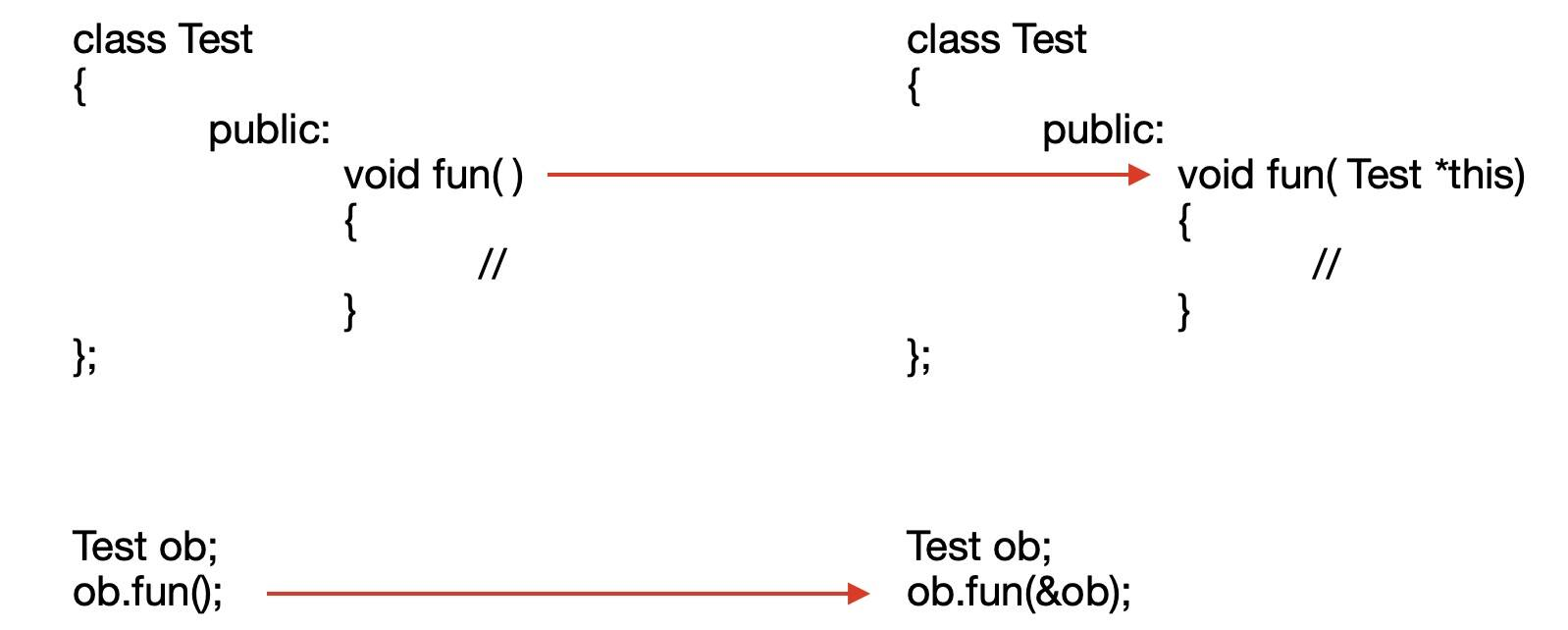const修饰成员函数后的this指针：

``Test::fun(const Test* const this){ // }``

const与指针：

``````int a = 5;
const int *p = &a;
*p = 10;//❌``````

``````int a = 5;
int b = 10;
int * const p = &a;
p = &b;//❌
*p = 10;//✅``````

``````int a = 5;
int b = 10;
const int * const p = &a;
*p = 10;//❌
p = &b;//❌``````

this指针的应用：

``````class Test
{
private:
int num;
public:
void setNum(int num)
{
this -> num = num;
}

};``````

``````class Test
{
public:
Test& Print(char *str)
{
cout << str;
return *this; // *this 等价 ob
}
}
int main()
{
Test ob;
ob.Print("h").Print("e").Print("l").Print("l").Print("o");
}``````

### 运算符重载

= [] () -> 必须重载成成员函数

``````returntype operator << (type name) { /function body/ }
ostream& operator << (ostream &out, Person &ob) { /function body/ }``````

cout << ob 等价operator << (cout, ob)

``````#include <iostream>
using namespace std;
class Data {
private:
int a;
int b;
public:
Data();
Data operator ++();
Data operator ++(int);
friend ostream& operator << (ostream &os, Data &ob);
};
Data::Data():a(0),b(0){ }
Data Data::operator ++ () {//重载前置自增
this -> a++;
this -> b++;
return *this;
}
Data Data::operator ++ (int) {//重载后置自增
Data tmp = *this;
this -> a++;
this -> b++;
return tmp;
}
ostream& operator << (ostream &os, Data &ob) {
os << "a = " << ob.a << " b = " << ob.b << endl;
return os;
}
int main() {
Data x;
++x;
cout << x;//a = 1 b = 1
Data y = x++;
cout << y;//a = 1 b = 1
cout << x;//a = 2 b = 2
return 0;
}``````

``````#include <iostream>
using namespace std;
class test {
private:
char *name;
public:
test();
test(const char* name);
~test();
};
test::test() : name(nullptr) { }
test::test(const char* name) {
this -> name = new char[strlen(name) + 1];
strcpy(this -> name, name);
}
test::~test() {
if (this -> name) {
delete []this -> name;
}
}
int main() {
test a("a");
test b = a;
}``````

test b = a;这里是旧对象给新对象赋值,调用的是拷贝构造,由于我们没有实现拷贝构造,调用的系统拷贝构造是单纯的赋值,会导致a的name成员和b的name成员共享一块内存,当a和b调用析构函数的时候就会释放已经释放的空间,导致错误

``````//只需要实现深拷贝即可
test::test(const test &ob) {
this -> name = new char[strlen(ob.name) + 1];
strcpy(this -> name, ob.name);
}``````

``````test a("a");
test b;
b = a;//❌ 旧对象给旧对象赋值``````
``````//重载 "=" 运算符
class test {
private:
char *name;
public:
void operator = (const test &ob);
//...
};
void test::operator = (const test &ob) {
this -> name = new char[strlen(ob.name) + 1];
strcpy(this -> name, ob.name);
}
//...``````

``````#include <iostream>
using namespace std;
class test {
private:
int i;
public:
test(int num);
test operator + (const test &ob);
bool operator && (const test &ob);
};
test::test(int num) : i(num) { }

bool test::operator && (const test &ob) {
return this -> i && ob.i;
}
test test::operator + (const test &ob) {
cout << "+" << endl;
test ret(0);
ret.i = this -> i + ob.i;
return ret;
}
int main() {
int a = 0, b = 1;
if (a && (a + b)) {
cout << "true" << endl;
} else {
cout << "false" << endl;
}
test obja(0), objb(1);
if (obja && (obja + objb)) {
cout << "true" << endl;
} else {
cout << "false" << endl;
}
return 0;
}
``````

### lambda表达式

lambda表达式是C++11的新特性，lambda表达式可以理解为内嵌的匿名函数，和普通函数类似，一个lambda具有一个返回类型、一个参数列表、一个函数体，并且lambda表达式可以定义在函数内部。

lambda表达式的形式如下:

``[capture list] (parameter list) -> return type { function body }``

capture list(捕获列表)，如果lambda表达式要使用所在函数内部的变量，就必须显式或隐式地在捕获列表声明，捕获列表可以为空。注意参数列表和返回类型可以省略，但捕获列表和函数体必须存在(即使为空)。

``auto f = [] { return 42; };``

lambda的捕获列表

[] 空捕获列表。lambda不能使用所在函数中的变量
[names] 捕获列表。默认为拷贝捕获
[&] 隐式捕获。采用引用捕获lambda所在函数的所有变量
[=] 隐式捕获。采用拷贝捕获lambda所在函数的所有变量
[&, names] 混合捕获。除了names以外的都采用引用捕获
[=, names] 混合捕获。除了names以外的都采用拷贝捕获

``````[&, &a, &b, &c]❌
[&, =a, =b, =c]✅``````

lambda是函数对象

``for_each(vec.cbegin(), vec.cend(), [](int val) { cout << val << " ";});``

for_each第三个参数为lambda表达式，其类似一个类的匿名对象，默认情况下lambda表达式不能改变它捕获的变量，所以重载函数调用运算符的函数是const的

，可以将lambda声明为mutable

``````class {
public:
void operator ()(int val) const { cout << val << " "; }
};``````

lambda表达式产生的类不含默认构造函数，赋值运算符(无法赋值但可以使用拷贝构造函数)及默认析构函数。

### 动态内存&智能指针

C++中使用new&delete/malloc&free来管理动态内存，但动态内存的使用容易出现问题：1.内存泄漏 2.使用空悬指针 3.对已释放的内存进行释放

``````void test() {
int *p = new int(5);
}
int main() {
test();
}``````

shared_ptr

shared_ptr允许多个指针指向同一个对象。

``````shared_ptr<string> p1;//指向stirng，默认为空
shared_ptr<int> p2;//指向int，默认为空``````

shared_ptr&unique_ptr都支持的操作

shared_ptr sp 空的shared指针，指向类型T
unique_ptr up 空的unique指针，指向类型T
p p指向对象则为空，否则为NULL
*p 解引用p，获得对象
p -> mem *等价(p).mem
p.get() 返回一个内置指针
swap(p, q) 交换指针
p.swap(q) 交换指针

shared_ptr独有的操作

make_shared(args) 返回一个shared_ptr，使用args初始化此对象
shared_ptr p(q) p为q的拷贝,增加q中的计数器
p = q 此操作递减q的引用计数，递增p的引用计数
p.unique() 若p.use_count()为1则为true否则为false
p.use_count() 返回与p共享对象的智能指针数量
shared_ptr p(q) p管理内置指针q所指的对象，p销毁时空间也将被释放
shared_ptr p(u) p从unique_ptr接管对象的所有权，将u置NULL
p.reset() 如果shared_ptr的use_count = 1，reset将会释放此对象，并且如果传递了可选参数内置指针q，会让p指向q，否则将p置NULL，并且可以传递删除器

shared_ptr会自动销毁对象和释放内存

``````shared_ptr<int> p1(make_shared<int>(5)); //p1.used_count() = 1
shared_ptr<int> p2(p1);
//p1.used_count() = 2 p2.used_count() = 2``````

``````shared_ptr<int> p1 = new int(1024);//❌
shared_ptr<int> p2(new int(1024));//✅``````

``````void func(shared_ptr<int> ptr) { // }
int main() {
int *p = new int(1024);
func(shared_ptr<int> (p));
auto x = *p;//❌
}``````

``````auto sp = make_shared<int>();
auto p = sp.get();
delete p;``````

get函数

get函数返回一个内置类型指针，但要注意：

1.使用get返回的指针不能delete空间，因为这块空间时归智能指针管理的

2.不能使用get返回的指针来为智能指针初始化或赋值

``````shared_ptr<int> p(new int(5));
int *q = p.get();
{ //新语句块
shared_ptr<int> tmp(q);
}
// p成为空悬指针``````

``````int x = 5;
shared_ptr<int> sp(&x, [](int *p) { *p = 0;});``````

unique_ptr管理删除器的方式与shared_ptr不同，我们必须在尖括号中unique_ptr指明类型之后，指明删除器类型(类似关联容器重载比较方式)

``````void helper(int *p) {
*p = 0;
}
int main() {
int x = 5;
using F = void (int*);
unique_ptr<int, F*> up(&x, helper);
}``````

``````void func() {
int *p = new int(5);
/*
使用p
*/
delete p;
}``````

unique_ptr

unique_ptr独享它所指向的对象，当unique_ptr被销毁时，它所指向的对象也将被释放，不可以拷贝或赋值unique_ptr,其拷贝构造和赋值函数声明为了delete。

unique_ptr的初始化方式类似shared_ptr，注意make_unique为C++14的新特性。

unique_ptr一些独有操作

unique u 空unique_ptr
unique<T,D> u(d) 空unique_ptr,用类型D的对象d来代替默认delete操作
u = nullptr 置空
u.release() u放弃对指针的控制权，返回指针并置空
u.reset() 释放u所指的对象，可提供可选参数传递一个内置指针q，令其指向q

``````unique<int[]> up(new int);
up.release();//自动调用delete[]
//
int *q = new int;
unique<int[]> up2(q);``````

unique_ptr管理数组时，不能使用点和箭头成员运算符，可以使用下标运算符

``````for (size_t i = 0 ; i < 10 ; ++i) {
up[i] = i;
}``````

``````shared_ptr<int> sp(new int, [](int *p){ delete []p;});
for (size_t i = 0 ; i != 10 ; ++i) {
*(sp.get() + i) = i;
}
sp.reset();``````

### 继承

###### 派生类定义格式:
``class 派生类名 : 继承方式 基类名 { }``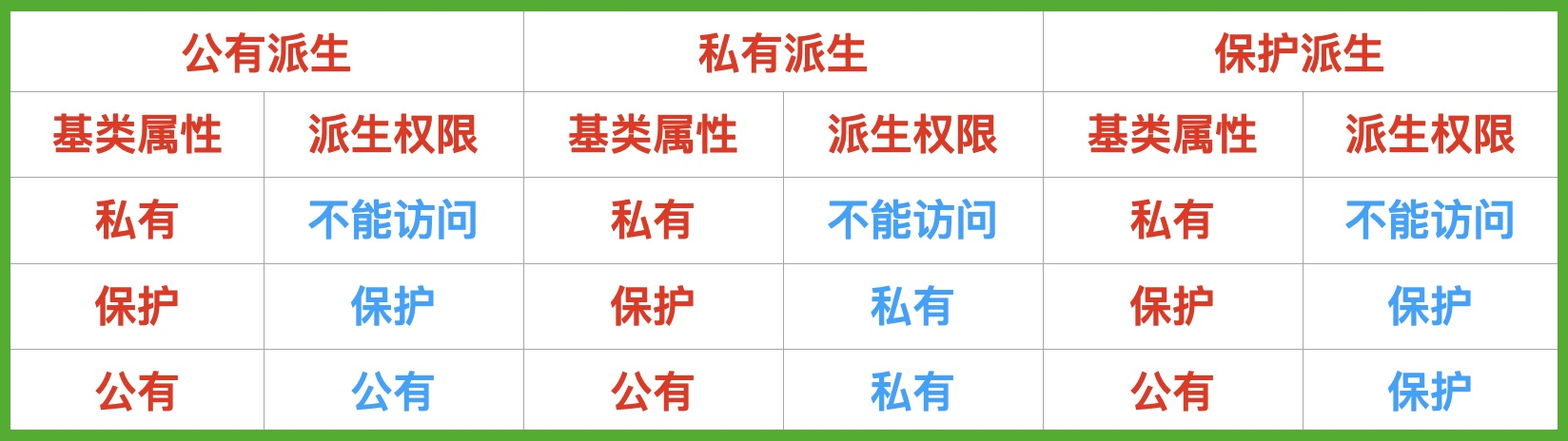1.不管是什么继承，父类中的private成员在子类都不可见(不管是在子类的外部还是内部)

2.公有派生保持不变，私有派生变为私有,保护派生变为保护

###### protected

protected位于public和private之间，当派生类继承基类后，继承的public成员类内类外都可访问，private成员都不可见，而protected对于派生类类内是可访问的(对对象不可访问)。

###### 访问说明符是对上层的说明

``````#include <iostream>
class A {
public:
int pub_m;
protected:
int pro_m;
private:
int pri_m;
};

class B : private A {

};

class C : public B {

};
int main() {
C c;
std::cout << c.pro_m << std::endl;//错误
return 0;
}``````
###### 继承和友元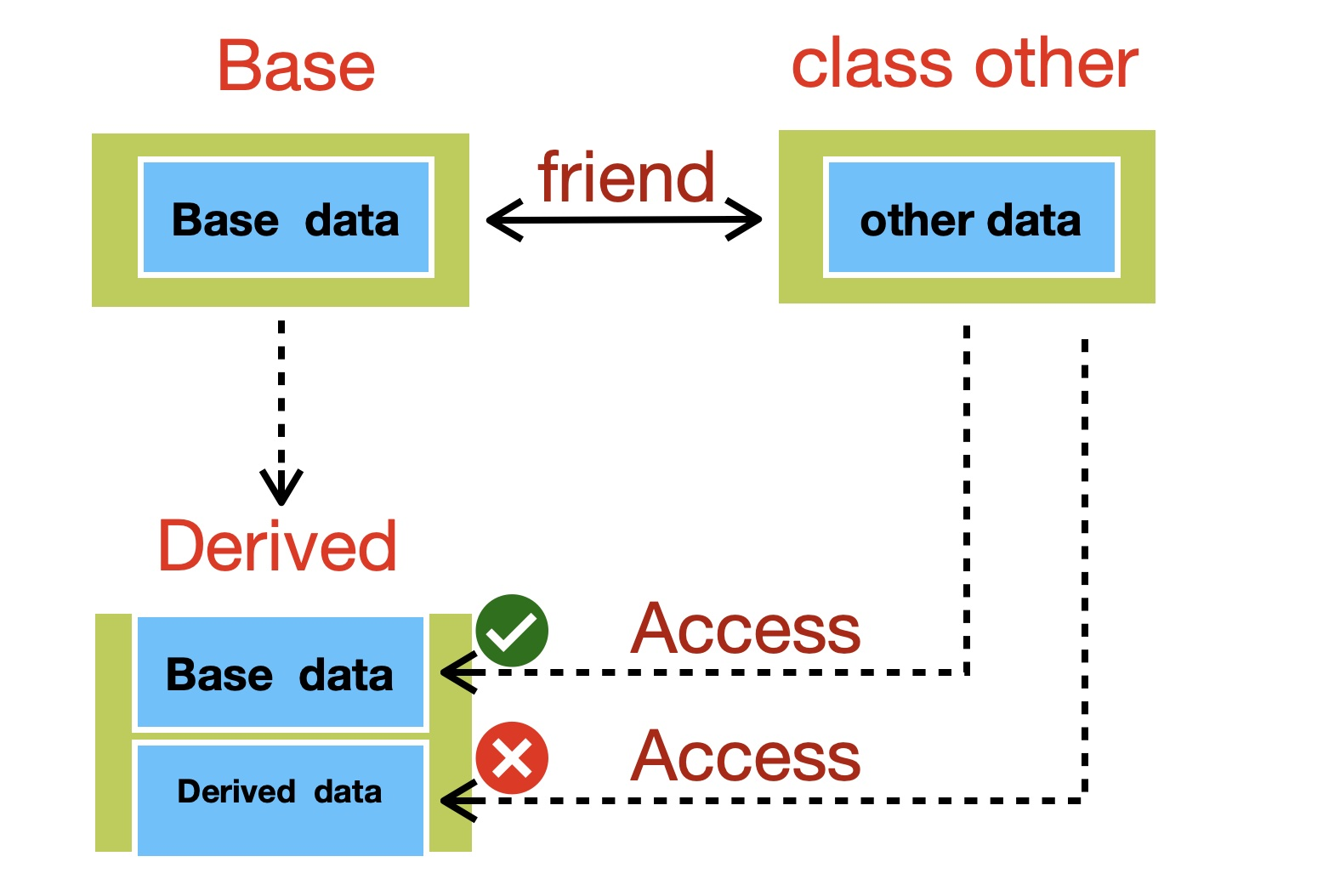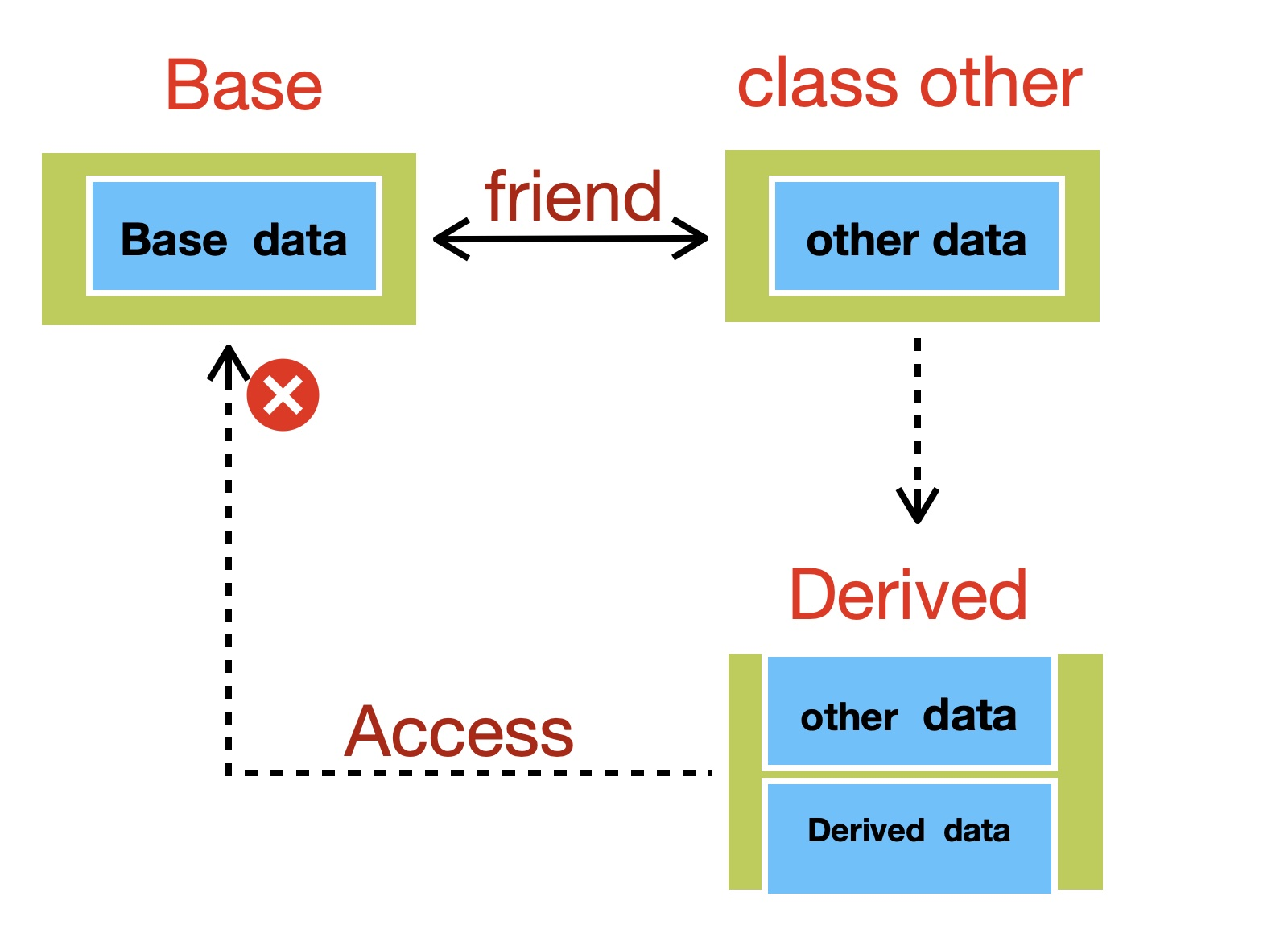###### 改变成员的可访问性

``````#include <iostream>
class Base {
public:
int a;
private:
int b;
protected:
int c;
};

class Derived : private {
public:
using Base::a;
using Base::c;
using Base::b;//错误,b在基类中为private，是不可见的，无法使用using
};
int main() {
Derived d;
std::cout << d.a << " " << d.c << std::endl;
//a和c的可访问性被修改为public,因此可以通过对象访问
return 0;
}``````
###### 类和class的区别

1.class的默认访问权限为private，struct则为public

2.class的默认继承为private，struct则为public

###### 派生类中的构造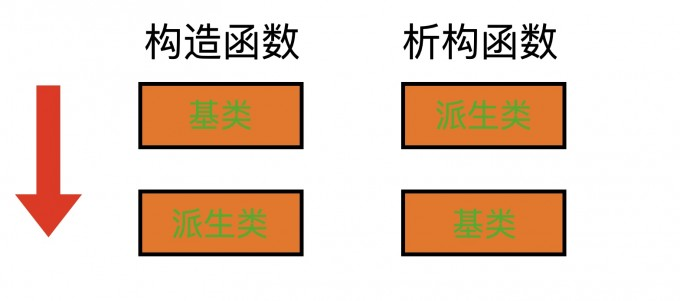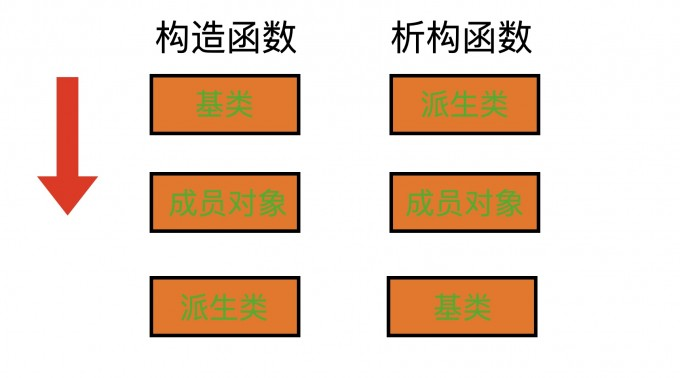###### 基类和派生类的类型转换
• 派生类向基类的类型转换只对指针或引用有效

• 不存在从基类向派生类的隐式转换

• 派生类向基类的转换不一定是有效的

``Base *p = new Derived;``

``````Base *p;//p的静态类型为Base
p = new Base;//p的动态类型为Base
p = new Derived;//p的动态类型为Derived``````

``````Derived *p = new Base;//不合法
Base *p = new Derived;
Derived *q = p;//不合法
q = static_cast<Derived*>(p);//强制转换``````

###### final & override关键字

``````class Base {
public:
virtual void fun1(int);
virtual void fun2();
void fun3();
};

class Derived : public Base {
public:
void fun1(int); override;//正确，有对应的虚函数需要重写
void fun2(double); override;//错误，没有形参为double的虚函数
void fun3(); override;//错误，fun3不为虚函数
};``````

final关键字可以作用于类或函数

``````class Base final {
//...
};
class Derived : public Base { //... }//错误``````

``````class A {
public:
virtual void fun(int);
};

class B : public A {
public:
virtual void fun(int) final;//正确，重写虚函数fun
};

class C : public B {
public:
virtual void fun(int);//错误，fun被声明为final了
};``````
###### 虚函数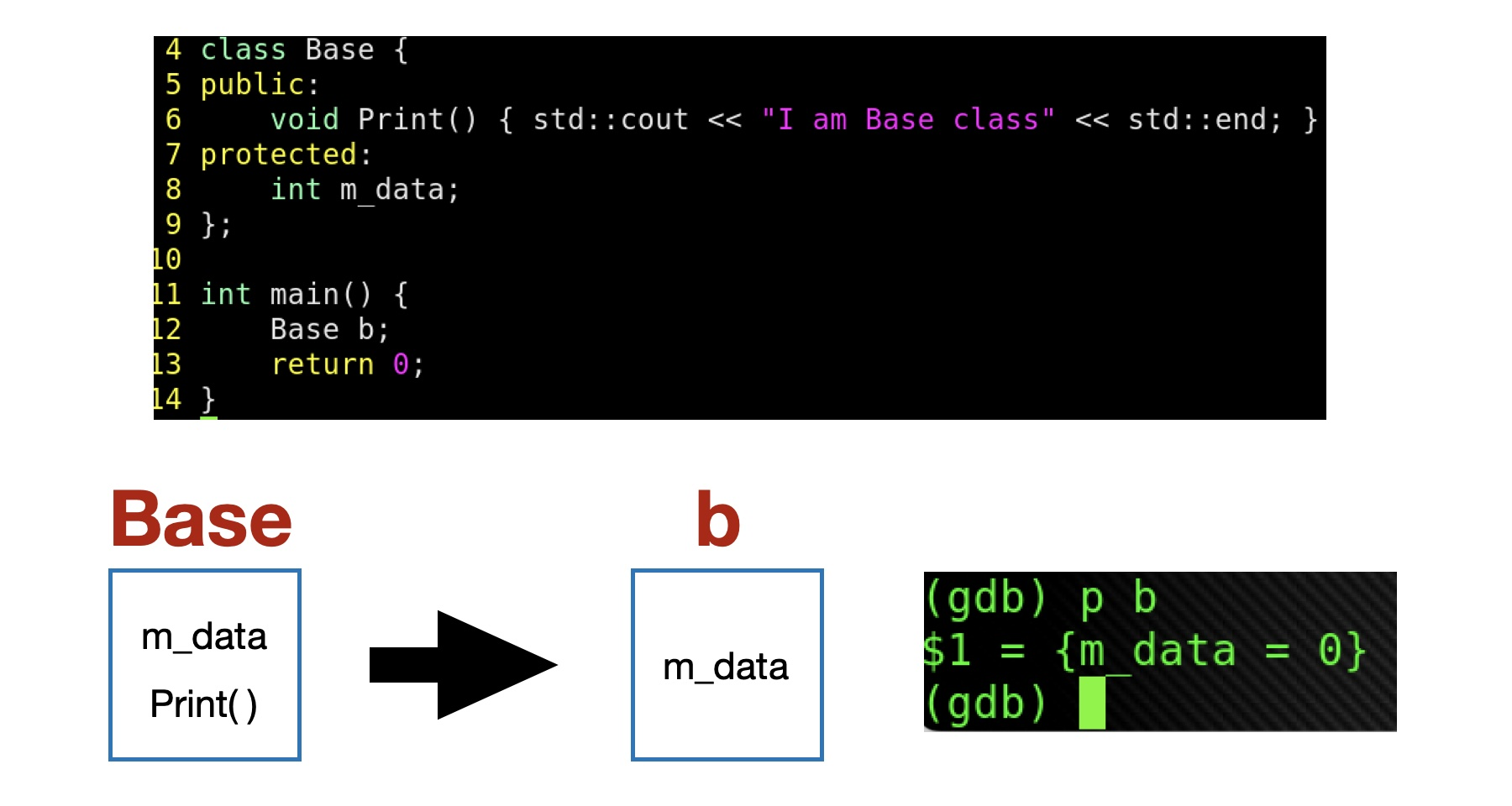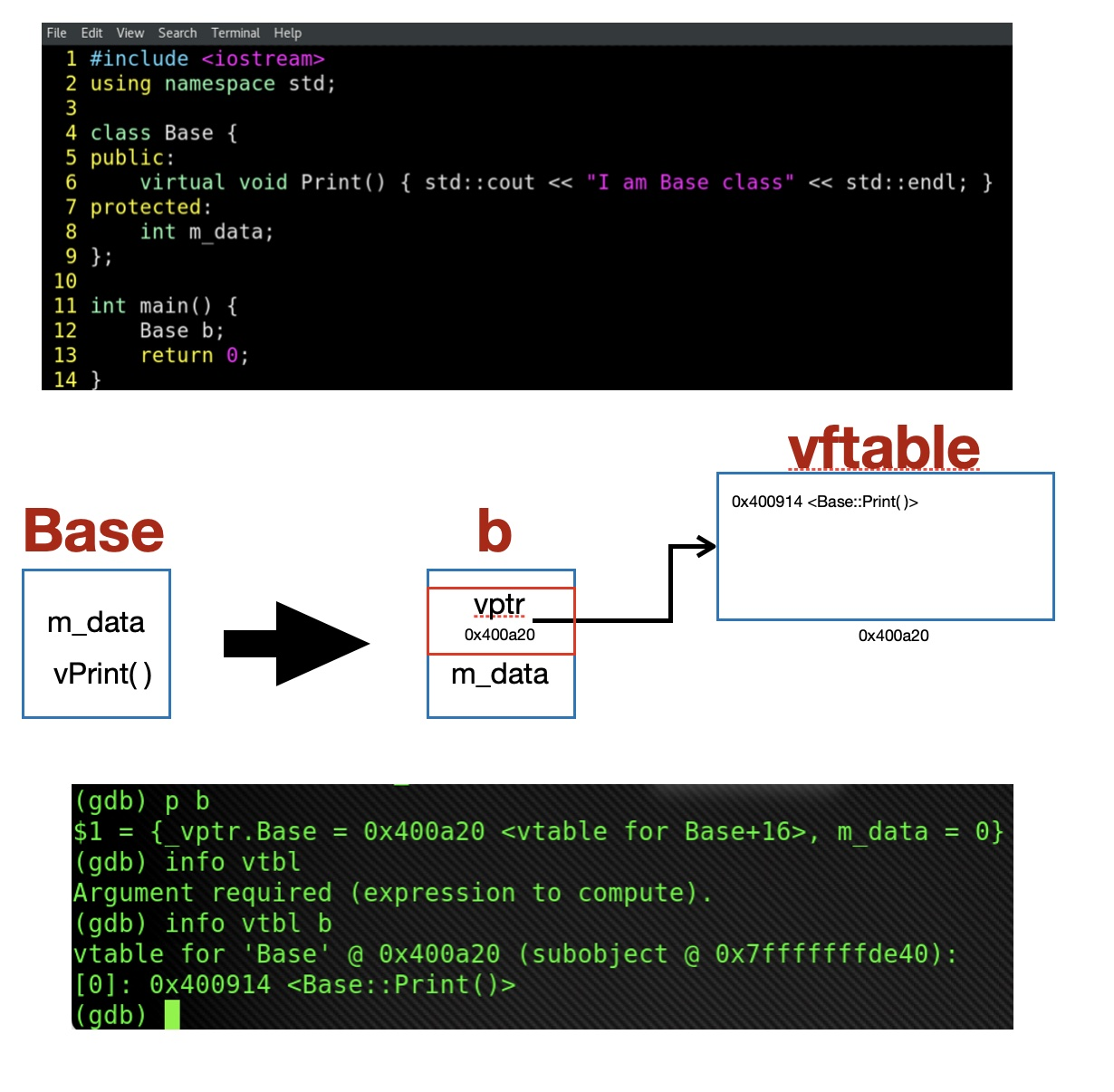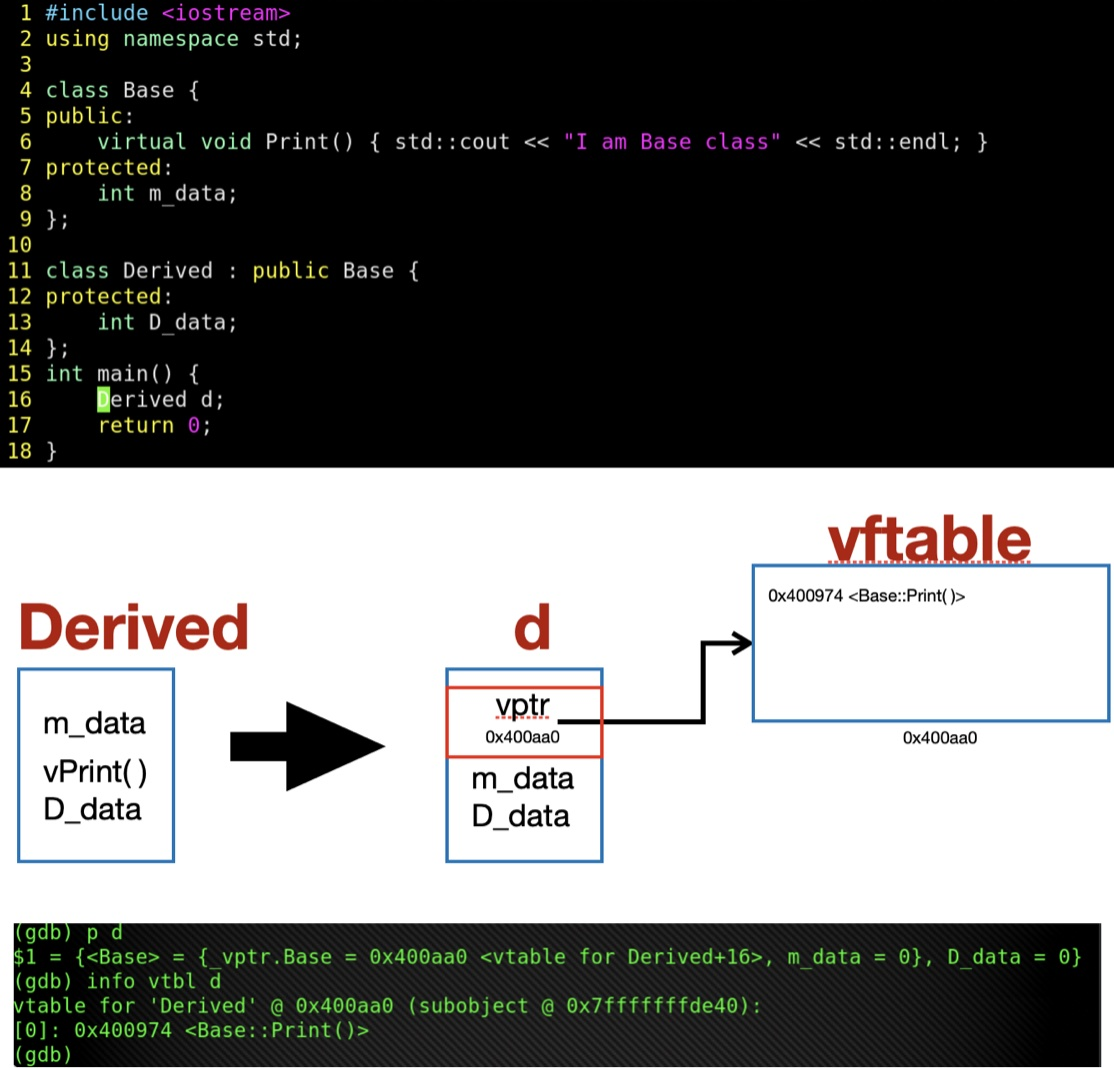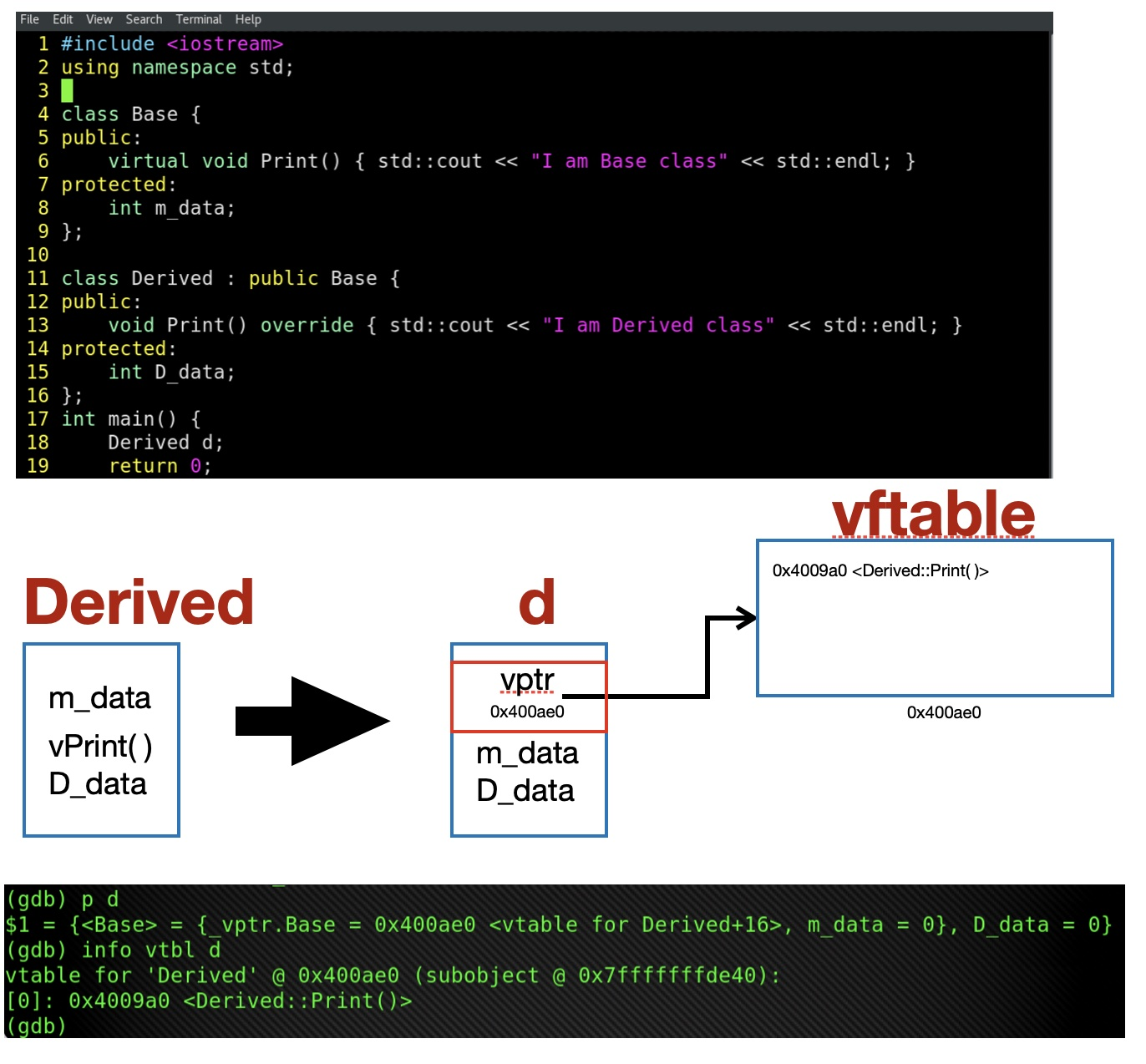``virtual void func() = 0;``

### 模板&泛型

###### 模版和重载

``````//版本1
template <typename T>
void func(const T &t) {}

//版本2
template <typename T>
void func(T *p) {}

string s("string");
func(s);``````

``````const string *p = &s;
func(p);``````

``````//版本3
void func(const string &s) {}``````

###### 模版特例化

``````//版本一
template <typename T>
int compare(const T &a, const T &b) {
if (a < b) return -1;
if (b < a) return 1;
return 0;
}``````

``````//版本二
template <size_t N, size_t M>
int compare(const char(&a)[N], const char(&b)[M]) {
return strcmp(a, b);
}``````

template后接一个空<>表示特例化，特例化表明我们会去指定类型，为原模板的所有模板参数都提供实参：

``````//版本三 特例化版本
template<>
int compare(const char* const &a, const char* const &b) {
return strcmp(a, b);
}``````

目录